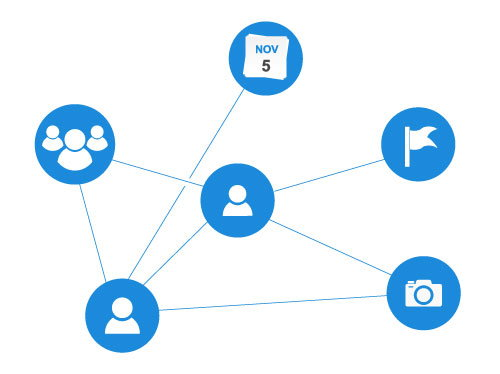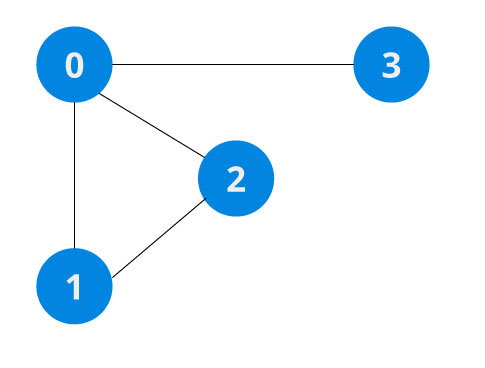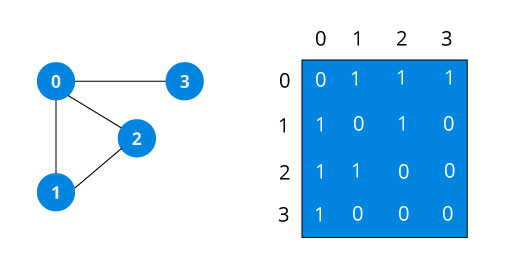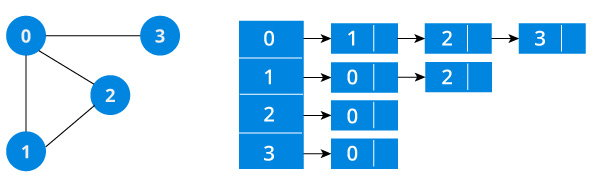# Graph Data Stucture

A graph data structure is a collection of nodes that have data and are connected to other nodes.

Let's try to understand this by means of an example. On facebook, everything is a node. That includes User, Photo, Album, Event, Group, Page, Comment, Story, Video, Link, Note...anything that has data is a node.

Every relationship is an edge from one node to another. Whether you post a photo, join a group, like a page etc., a new edge is created for that relationship.All of facebook is then, a collection of these nodes and edges. This is because facebook uses a graph data structure to store its data.

More precisely, a graph is a data structure (V,E) that consists of

• A collection of vertices V
• A collection of edges E, represented as ordered pairs of vertices (u,v)In the graph,

```V = {0, 1, 2, 3}
E = {(0,1), (0,2), (0,3), (1,2)}
G = {V, E}```

## Graph Terminology

• Adjacency: A vertex is said to be adjacent to another vertex if there is an edge connecting them. Vertices 2 and 3 are not adjacent because there is no edge between them.
• Path: A sequence of edges that allows you to go from vertex A to vertex B is called a path. 0-1, 1-2 and 0-2 are paths from vertex 0 to vertex 2.
• Directed Graph: A graph in which an edge (u,v) doesn't necessary mean that there is an edge (v, u) as well. The edges in such a graph are represented by arrows to show the direction of the edge.

## Graph Representation

Graphs are commonly represented in two ways:

An adjacency matrix is 2D array of V x V vertices. Each row and column represent a vertex.

If the value of any element `a[i][j]` is 1, it represents that there is an edge connecting vertex i and vertex j.

The adjacency matrix for the graph we created above isSince it is an undirected graph, for edge (0,2), we also need to mark edge (2,0); making the adjacency matrix symmetric about the diagonal.

Edge lookup(checking if an edge exists between vertex A and vertex B) is extremely fast in adjacency matrix representation but we have to reserve space for every possible link between all vertices(V x V), so it requires more space.

An adjacency list represents a graph as an array of linked list.

The index of the array represents a vertex and each element in its linked list represents the other vertices that form an edge with the vertex.

The adjacency list for the graph we made in the first example is as follows:An adjacency list is efficient in terms of storage because we only need to store the values for the edges. For a graph with millions of vertices, this can mean a lot of saved space.

## Graph Operations

The most common graph operations are:

• Check if element is present in graph
• Graph Traversal
• Add elements(vertex, edges) to graph
• Finding path from one vertex to another